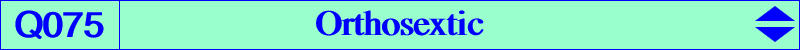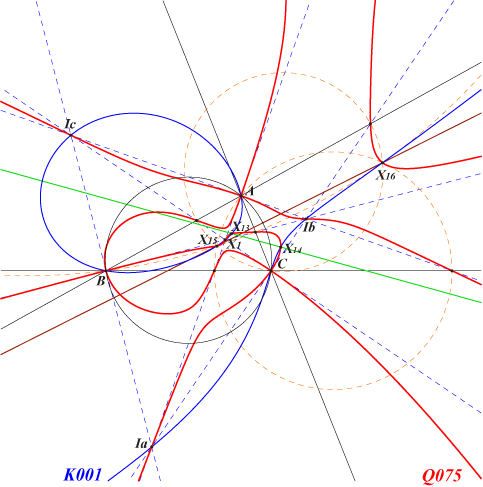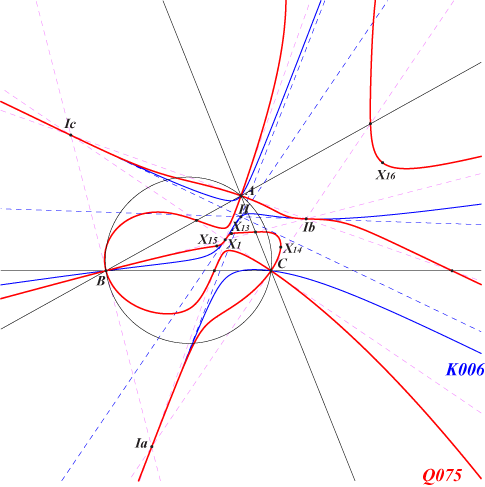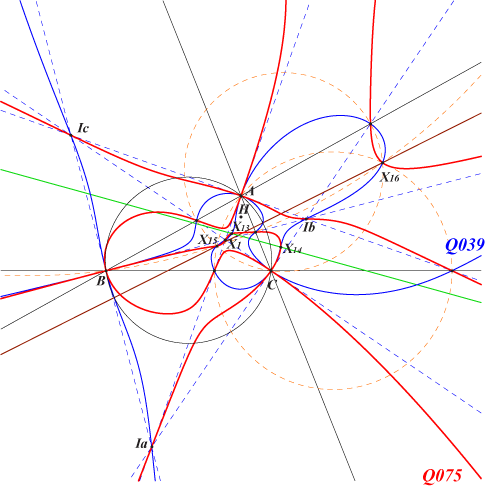see belowX(1), X(13), X(14), X(15), X(16) excenters, feet of bisectors Ix-anticevian points, see Table 23 isogonal conjugates of the Ix-anticevian points, see Table 23 common points of (O) and the complement of the Jerabek hyperbolaQ075 is the locus of point P such that the anticevian triangle of P is perspective to the reflection triangle of the isogonal conjugate P* of P. See Table 6. Q075 is a circular isogonal sextic with three nodes at A, B, C. The nodal tangents are the bisectors of ABC. The equation of Q075 can be written under the form : 4xyz ∑SB SC x (c2y2- b2z2) + ∏(c2y2- b2z2) = 0, where ∑SB SC x (c2y2- b2z2) = 0 is the equation of the Orthocubic K006 and ∏(c2y2- b2z2) = 0 is that of the six bisectors of ABC. It follows that the tangents at the in/excenters to Q075 pass through the orthocenter H of ABC.Q075 and the Orthocubic K006.Q075 is very similar to the sextic Q039 since the Orthocubic is replaced by the Darboux cubic K004. These two sextics generate a pencil which also contains Q076, the analogous sextic associated with the Napoleon cubic K005.